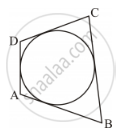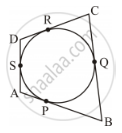Share

# In Figure 3, a Circle Touches All the Four Sides of a Quadrilateral Abcd Whose Sides Are Ab = 6 Cm, Bc = 9 Cm and Cd = 8 Cm. Find the Length of the Side Ad. - CBSE Class 10 - Mathematics

ConceptCircles Examples and Solutions

#### Question

In Figure 3, a circle touches all the four sides of a quadrilateral ABCD whose sides are AB = 6 cm, BC = 9 cm and CD = 8 cm. Find the length of the side AD.#### Solution

Let the inscribed circle touch sides AB, BC, CD and DA of quadrilateral ABCD at P, Q, R and S respectively.It is known that the lengths of tangents drawn from an external point to a circle are equal.

∴ AS = AP, DS = DR, BP = BQ, CR = CQ

= AS + DS

= AP + DR

= (6 − PB) + (8 − CR)

= 14 cm − BQ − CQ [(Using (1)]

= 14 cm − (BQ + CQ)

= 14 cm − BC

= (14 − 9) cm

= 5 cm

Thus, the length of side AD is 5 cm

Is there an error in this question or solution?

#### Video TutorialsVIEW ALL 

Solution In Figure 3, a Circle Touches All the Four Sides of a Quadrilateral Abcd Whose Sides Are Ab = 6 Cm, Bc = 9 Cm and Cd = 8 Cm. Find the Length of the Side Ad. Concept: Circles Examples and Solutions.
S